# AKTU 1st Year Sem 1 Solved Paper 2014-15 | COMP. SYSTEM & C PROGRAMMING | Sec B

• Last Updated : 30 Jan, 2019

# B.Tech.(SEM-I) THEORY EXAMINATION 2014-15COMPUTER SYSTEM & PROGRAMMING IN C

Time: 3hrs
Total Marks: 100

Note:-

• Attempt all questions. Marks are indicated against each question.
• Assume suitable data wherever necessary.
• ### 2. Attempt any TWO parts: (10*2 = 20)

1. Describe about the basic components of computers with a neat block diagram.

Digital Computer: A digital computer can be defined as a programmable machine which reads the binary data passed as instructions, processes this binary data, and displays a calculated digital output. Therefore, Digital computers are those that work on the digital data.

Details of Functional Components of a Digital Computer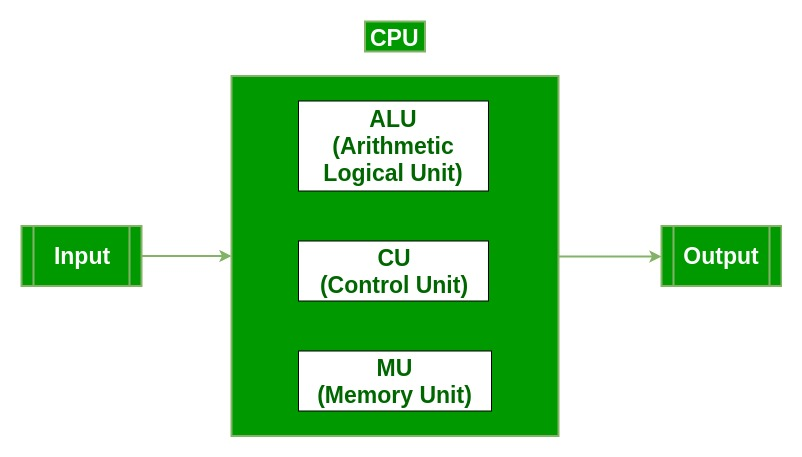• Input Unit :The input unit consists of input devices that are attached to the computer. These devices take input and convert it into binary language that the computer understands. Some of the common input devices are keyboard, mouse, joystick, scanner etc.
• Central Processing Unit (CPU0 : Once the information is entered into the computer by the input device, the processor processes it. The CPU is called the brain of the computer because it is the control center of the computer. It first fetches instructions from memory and then interprets them so as to know what is to be done. If required, data is fetched from memory or input device. Thereafter CPU executes or performs the required computation and then either stores the output or displays on the output device. The CPU has three main components which are responsible for different functions – Arithmetic Logic Unit (ALU), Control Unit (CU) and Memory registers
• Arithmetic and Logic Unit (ALU) : The ALU, as its name suggests performs mathematical calculations and takes logical decisions. Arithmetic calculations include addition, subtraction, multiplication and division. Logical decisions involve comparison of two data items to see which one is larger or smaller or equal.
• Control Unit : The Control unit coordinates and controls the data flow in and out of CPU and also controls all the operations of ALU, memory registers and also input/output units. It is also responsible for carrying out all the instructions stored in the program. It decodes the fetched instruction, interprets it and sends control signals to input/output devices until the required operation is done properly by ALU and memory.
• Memory Registers : A register is a temporary unit of memory in the CPU. These are used to store the data which is directly used by the processor. Registers can be of different sizes(16 bit, 32 bit, 64 bit and so on) and each register inside the CPU has a specific function like storing data, storing an instruction, storing address of a location in memory etc. The user registers can be used by an assembly language programmer for storing operands, intermediate results etc. Accumulator (ACC) is the main register in the ALU and contains one of the operands of an operation to be performed in the ALU.
• Memory : Memory attached to the CPU is used for storage of data and instructions and is called internal memory The internal memory is divided into many storage locations, each of which can store data or instructions. Each memory location is of the same size and has an address. With the help of the address, the computer can read any memory location easily without having to search the entire memory. when a program is executed, it’s data is copied to the internal memory ans is stored in the memory till the end of the execution. The internal memory is also called the Primary memory or Main memory. This memory is also called as RAM, i.e. Random Access Memory. The time of access of data is independent of its location in memory, therefore this memory is also called Random Access memory (RAM). Read this for different types of RAMs
• Output Unit : The output unit consists of output devices that are attached with the computer. It converts the binary data coming from CPU to human understandable form. The common output devices are monitor, printer, plotter etc.
2. Explain logical and bit operators with example.

• Logical Operators:  Logical Operators are used to combine two or more conditions/constraints or to complement the evaluation of the original condition in consideration. The result of the operation of a logical operator is a boolean value either true or false. To learn about different logical operators in details please visit this link.

They are used to combine two or more conditions/constraints or to complement the evaluation of the original condition in consideration. They are described below:

• Logical AND: The ‘&&’ operator returns true when both the conditions in consideration are satisfied. Otherwise it returns false. For example, a && b returns true when both a and b are true (i.e. non-zero).
• Logical OR: The ‘||’ operator returns true when one (or both) of the conditions in consideration is satisfied. Otherwise it returns false. For example, a || b returns true if one of a or b is true (i.e. non-zero). Of course, it returns true when both a and b are true.
• Logical NOT: The ‘!’ operator returns true the condition in consideration is not satisfied. Otherwise it returns false. For example, !a returns true if a is false, i.e. when a=0.

 `// C program to demonstrate working of logical operators``#include `` ` `int` `main()``{``    ``int` `a=10, b=4, c = 10, d = 20;`` ` `    ``// logical operators`` ` `    ``// logical AND example``    ``if` `(a>b && c==d)``        ``printf``(``"a is greater than b AND c is equal to d\n"``);``    ``else` `printf``(``"AND condition not satisfied\n"``);`` ` `    ``// logical AND example``    ``if` `(a>b || c==d)``        ``printf``(``"a is greater than b OR c is equal to d\n"``);``    ``else` `printf``(``"Neither a is greater than b nor c is equal "``                ``" to d\n"``);`` ` `    ``// logical NOT example``    ``if` `(!a)``        ``printf``(``"a is zero\n"``);``    ``else` `printf``(``"a is not zero"``);`` ` `    ``return` `0;``}`

Output:

```AND condition not satisfied
a is greater than b OR c is equal to d
a is not zero```
• Bitwise Operators: The Bitwise operators is used to perform bit-level operations on the operands. The operators are first converted to bit-level and then calculation is performed on the operands. The mathematical operations such as addition, subtraction, multiplication etc. can be performed at bit-level for faster processing. To learn bitwise operators in details, visit this link.

In C, following 6 operators are bitwise operators (work at bit-level)

• & (bitwise AND) Takes two numbers as operands and does AND on every bit of two numbers. The result of AND is 1 only if both bits are 1.
• | (bitwise OR) Takes two numbers as operands and does OR on every bit of two numbers. The result of OR is 1 any of the two bits is 1.
• ^ (bitwise XOR) Takes two numbers as operands and does XOR on every bit of two numbers. The result of XOR is 1 if the two bits are different.
• << (left shift) Takes two numbers, left shifts the bits of the first operand, the second operand decides the number of places to shift.
• >> (right shift) Takes two numbers, right shifts the bits of the first operand, the second operand decides the number of places to shift.
• ~ (bitwise NOT) Takes one number and inverts all bits of it

Following is example C program.

 `/* C Program to demonstrate use of bitwise operators */``#include``int` `main()``{``    ``unsigned ``char` `a = 5, b = 9; ``// a = 5(00000101), b = 9(00001001)``    ``printf``(``"a = %d, b = %d\n"``, a, b);``    ``printf``(``"a&b = %d\n"``, a&b); ``// The result is 00000001``    ``printf``(``"a|b = %d\n"``, a|b);  ``// The result is 00001101``    ``printf``(``"a^b = %d\n"``, a^b); ``// The result is 00001100``    ``printf``(``"~a = %d\n"``, a = ~a);   ``// The result is 11111010``    ``printf``(``"b<<1 = %d\n"``, b<<1);  ``// The result is 00010010 ``    ``printf``(``"b>>1 = %d\n"``, b>>1);  ``// The result is 00000100 ``    ``return` `0;``}`

Output:

```a = 5, b = 9
a&b = 1
a|b = 13
a^b = 12
~a = 250
b<<1 = 18
b>>1 = 4
```
3. Write about the formatted and unformatted input/output functions in C.

Formatted Output means changing the output pattern in a C language as per the specifications. It is done using Format Specifiers in C. Format specifier is used during input and output. It is a way to tell the compiler what type of data is in a variable during taking input using scanf() or printing using printf(). Some examples are %c, %d, %f, etc.

Examples:

 `#include ``int` `main()``{``    ``int` `x = 45, y = 90;``    ``char` `ch = ``'A'``;``    ``printf``(``"%c\n"``, ch);``    ``printf``(``"%d\n"``, x);``    ``printf``(``"%i\n"``, x);``    ``return` `0;``}`

Output:

```A
45
45
```
4. ### 3. Attempt any TWO parts: (10*2 = 20)

1. Describe about the types of looping statements in C with necessary syntax

There are mainly two types of loops:

1. Entry Controlled loops: In this type of loops the test condition is tested before entering the loop body. For Loop and While Loop are entry controlled loops.
2. Exit Controlled Loops: In this type of loops the test condition is tested or evaluated at the end of loop body. Therefore, the loop body will execute atleast once, irrespective of whether the test condition is true or false. do – while loop is exit controlled loop.

for Loop

A for loop is a repetition control structure which allows us to write a loop that is executed a specific number of times. The loop enables us to perform n number of steps together in one line.
Syntax:

```for (initialization expr; test expr; update expr)
{
// body of the loop
// statements we want to execute
}
```

In for loop, a loop variable is used to control the loop. First initialize this loop variable to some value, then check whether this variable is less than or greater than counter value. If statement is true, then loop body is executed and loop variable gets updated . Steps are repeated till exit condition comes.

• Initialization Expression: In this expression we have to initialize the loop counter to some value. for example: int i=1;
• Test Expression: In this expression we have to test the condition. If the condition evaluates to true then we will execute the body of loop and go to update expression otherwise we will exit from the for loop. For example: i <= 10;
• Update Expression: After executing loop body this expression increments/decrements the loop variable by some value. for example: i++;

Equivalent flow diagram for loop :While Loop

While studying for loop we have seen that the number of iterations is known beforehand, i.e. the number of times the loop body is needed to be executed is known to us. while loops are used in situations where we do not know the exact number of iterations of loop beforehand. The loop execution is terminated on the basis of test condition.

Syntax:
We have already stated that a loop is mainly consisted of three statements – initialization expression, test expression, update expression. The syntax of the three loops – For, while and do while mainly differs on the placement of these three statements.

```initialization expression;
while (test_expression)
{
// statements

update_expression;
}
```

Flow Diagram: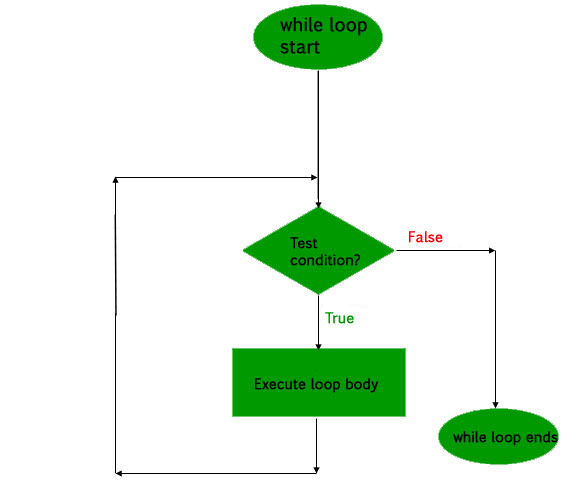do while loop

In do while loops also the loop execution is terminated on the basis of test condition. The main difference between do while loop and while loop is in do while loop the condition is tested at the end of loop body, i.e do while loop is exit controlled whereas the other two loops are entry controlled loops.
Note: In do while loop the loop body will execute at least once irrespective of test condition.

Syntax:

```initialization expression;
do
{
// statements

update_expression;
} while (test_expression);
```

Note: Notice the semi – colon(“;”) in the end of loop.

Flow Diagram: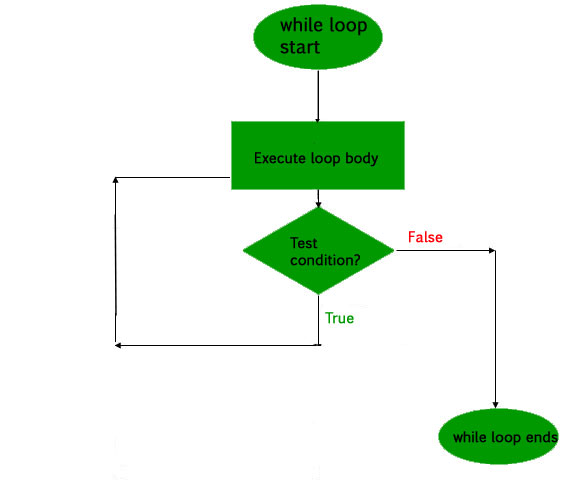2. Write a C program to find the multiplication of two matrices.

 `// C program to multiply two square matrices.`` ` `#include `` ` `const` `int` `MAX = 100;`` ` `// Function to print Matrix``void` `printMatrix(``int` `M[][MAX], ``int` `rowSize, ``int` `colSize)``{``    ``for` `(``int` `i = 0; i < rowSize; i++) {``        ``for` `(``int` `j = 0; j < colSize; j++)``            ``printf``(``"%d "``, M[i][j]);`` ` `        ``printf``(``"\n"``);``    ``}``}`` ` `// Function to multiply two matrices A[][] and B[][]``void` `multiplyMatrix(``int` `row1, ``int` `col1, ``int` `A[][MAX],``                    ``int` `row2, ``int` `col2, ``int` `B[][MAX])``{``    ``int` `i, j, k;`` ` `    ``// Matrix to store the result``    ``int` `C[MAX][MAX];`` ` `    ``// Check if multiplication is Possible``    ``if` `(row2 != col1) {``        ``printf``(``"Not Possible\n"``);``        ``return``;``    ``}`` ` `    ``// Multiply the two``    ``for` `(i = 0; i < row1; i++) {``        ``for` `(j = 0; j < col2; j++) {``            ``C[i][j] = 0;``            ``for` `(k = 0; k < row2; k++)``                ``C[i][j] += A[i][k] * B[k][j];``        ``}``    ``}`` ` `    ``// Print the result``    ``printf``(``"\nResultant Matrix: \n"``);``    ``printMatrix(C, row1, col2);``}`` ` `// Driven Program``int` `main()``{``    ``int` `row1, col1, row2, col2, i, j;``    ``int` `A[MAX][MAX], B[MAX][MAX];`` ` `    ``// Read size of Matrix A from user``    ``printf``(``"Enter the number of rows of First Matrix: "``);``    ``scanf``(``"%d"``, &row1);``    ``printf``(``"%d"``, row1);``    ``printf``(``"\nEnter the number of columns of First Matrix: "``);``    ``scanf``(``"%d"``, &col1);``    ``printf``(``"%d"``, col1);`` ` `    ``// Read the elements of Matrix A from user``    ``printf``(``"\nEnter the elements of First Matrix: "``);``    ``for` `(i = 0; i < row1; i++) {``        ``for` `(j = 0; j < col1; j++) {``            ``printf``(``"\nA[%d][%d]: "``, i, j);``            ``scanf``(``"%d"``, &A[i][j]);``            ``printf``(``"%d"``, A[i][j]);``        ``}``    ``}`` ` `    ``// Read size of Matrix B from user``    ``printf``(``"\nEnter the number of rows of Second Matrix: "``);``    ``scanf``(``"%d"``, &row2);``    ``printf``(``"%d"``, row2);``    ``printf``(``"\nEnter the number of columns of Second Matrix: "``);``    ``scanf``(``"%d"``, &col2);``    ``printf``(``"%d"``, col2);`` ` `    ``// Read the elements of Matrix B from user``    ``printf``(``"\nEnter the elements of First Matrix: "``);``    ``for` `(i = 0; i < row2; i++) {``        ``for` `(j = 0; j < col2; j++) {``            ``printf``(``"\nB[%d][%d]: "``, i, j);``            ``scanf``(``"%d"``, &B[i][j]);``            ``printf``(``"%d"``, B[i][j]);``        ``}``    ``}`` ` `    ``// Print the Matrix A``    ``printf``(``"\n\nFirst Matrix: \n"``);``    ``printMatrix(A, row1, col1);`` ` `    ``// Print the Matrix B``    ``printf``(``"\nSecond Matrix: \n"``);``    ``printMatrix(B, row2, col2);`` ` `    ``// Find the product of the 2 matrices``    ``multiplyMatrix(row1, col1, A, row2, col2, B);`` ` `    ``return` `0;``}`

Output:

```Enter the number of rows of First Matrix: 2
Enter the number of columns of First Matrix: 3
Enter the elements of First Matrix:
A: 1
A: 2
A: 3
A: 4
A: 5
A: 6

Enter the number of rows of Second Matrix: 3
Enter the number of columns of Second Matrix: 2
Enter the elements of First Matrix:
B: 1
B: 2
B: 3
B: 4
B: 5
B: 6

First Matrix:
1 2 3
4 5 6

Second Matrix:
1 2
3 4
5 6

Resultant Matrix:
22 28
49 64
```
3. What are the types of function? Write a C program to find the factorial of a given number using recursion.

There are following categories: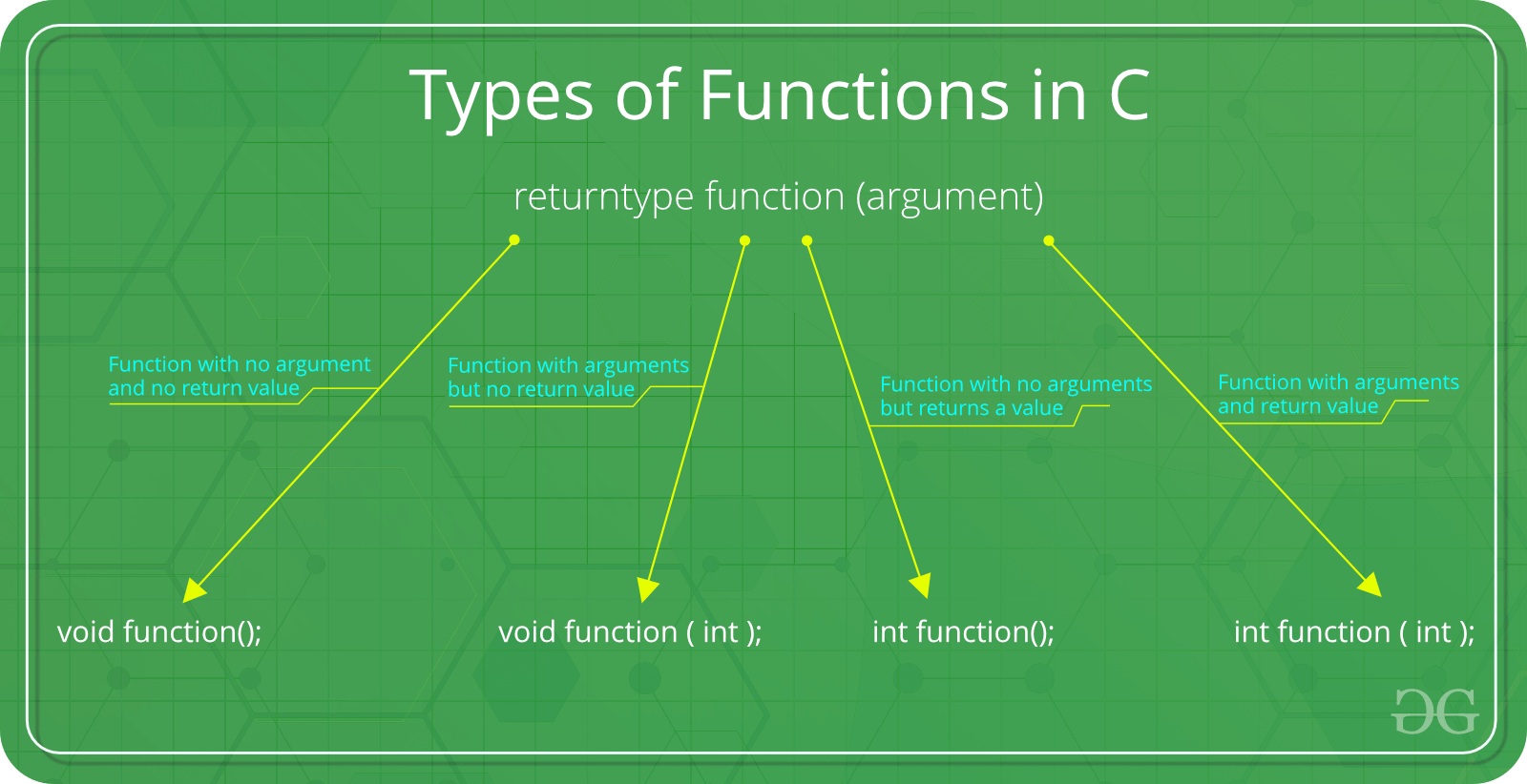1. Function with no argument and no return value : When a function has no arguments, it does not receive any data from the calling function. Similarly when it does not return a value, the calling function does not receive any data from the called function.
Syntax :

```Function declaration : void function();
Function call : function();
Function definition :
void function()
{
statements;
}
```
2. Function with arguments but no return value : When a function has arguments, it receive any data from the calling function but it returns no values.

Syntax :

```Function declaration : void function ( int );
Function call : function( x );
Function definition:
void function( int x )
{
statements;
}
```
3. Function with no arguments but returns a value : There could be occasions where we may need to design functions that may not take any arguments but returns a value to the calling function. A example for this is getchar function it has no parameters but it returns an integer an integer type data that represents a character.
Syntax :

```Function declaration : int function();
Function call : function();
Function definition :
int function()
{
statements;
return x;
}
```
4. Function with arguments and return value
Syntax :

```Function declaration : int function ( int );
Function call : function( x );
Function definition:
int function( int x )
{
statements;
return x;
}
```

Program to find factorial of a number:

 `// C program to find factorial of given number``#include `` ` `// function to find factorial of given number``unsigned ``int` `factorial(unsigned ``int` `n)``{`` ` `    ``// Base case``    ``if` `(n == 0)``        ``return` `1;`` ` `    ``// Recursively call factorial function``    ``return` `n * factorial(n - 1);``}`` ` `int` `main()``{`` ` `    ``int` `num;`` ` `    ``// Ge the number of which``    ``// factorial is to be calculated``    ``scanf``(``"%d"``, &num);``    ``printf``(``"Enter the number: %d"``, num);`` ` `    ``// Find the factorial``    ``// and print the result``    ``printf``(``"\nFactorial of %d is %d"``,``           ``num, factorial(num));`` ` `    ``return` `0;``}`

Output:

```Enter the number: 5
Factorial of 5 is 120```

### 4. Attempt any TWO parts: (10*2 = 20)

1. How to declare an array? Explain about various operations of an array.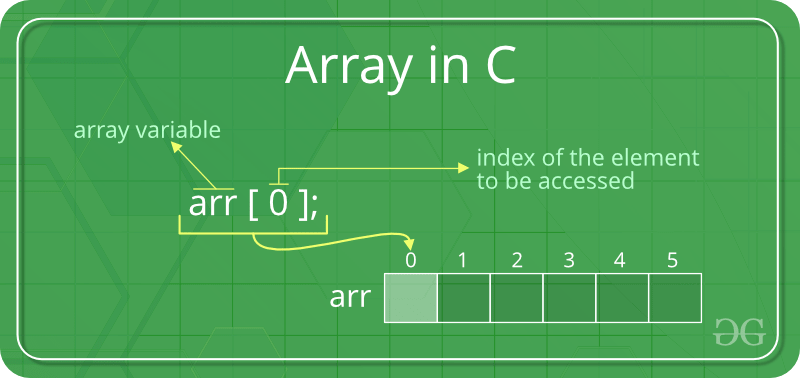Examples to declare the array

```// A character array in C/C++/Java
char arr1[] = {'g', 'e', 'e', 'k', 's'};

// An Integer array in C/C++/Java
int arr2[] = {10, 20, 30, 40, 50};

// Item at i'th index in array is typically accessed
// as "arr[i]".  For example arr1 gives us 'g'
// and arr2 gives us 40.
```

For operations on Array:

2. What is enumerated data type? Write a C program to display months in the year using enum.

Enumerated Data-type: Enumeration (or enum) is a user defined data type in C. It is mainly used to assign names to integral constants, the names make a program easy to read and maintain.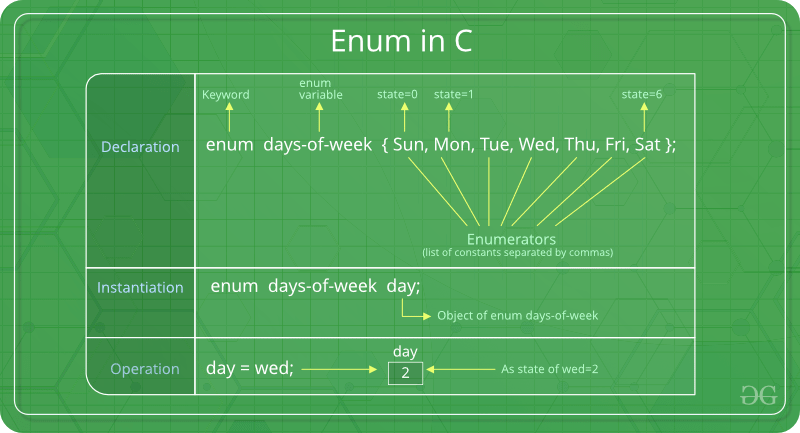`enum State {Working = 1, Failed = 0}; `

The keyword ‘enum’ is used to declare new enumeration types in C and C++.

Following is an example of enum declaration.

```// The name of enumeration is "flag" and the constant
// are the values of the flag. By default, the values
// of the constants are as follows:
// constant1 = 0, constant2 = 1, constant3 = 2 and
// so on.
enum flag{constant1, constant2, constant3, ....... };
```

Variables of type enum can also be defined. They can be defined in two ways:

```// In both of the below cases, "day" is
// defined as the variable of type week.

enum week{Mon, Tue, Wed};
enum week day;

// Or

enum week{Mon, Tue, Wed}day;
```

C program to display months in the year using enum:

 `// Another example program to demonstrate working``// of enum in C``#include `` ` `enum` `year { Jan,``            ``Feb,``            ``Mar,``            ``Apr,``            ``May,``            ``Jun,``            ``Jul,``            ``Aug,``            ``Sep,``            ``Oct,``            ``Nov,``            ``Dec };`` ` `int` `main()``{``    ``int` `i;``    ``for` `(i = Jan; i <= Dec; i++)``        ``printf``(``"%d "``, i);`` ` `    ``return` `0;``}`

Output:

```0 1 2 3 4 5 6 7 8 9 10 11
```

In this example, the for loop will run from i = 0 to i = 11, as initially the value of i is Jan which is 0 and the value of Dec is 11.

3. Differentiate structure and union in C.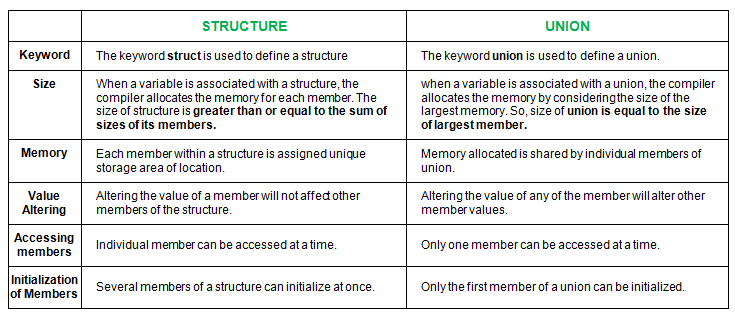### 5. Attempt any TWO parts: (10*2 = 20)

1. State the features of pointers. Write a C program to sort a given number using pointers.

Features Of Pointers:

1. Pointers save memory space.
2. Execution time with pointers is faster, because data are manipulated with the address, that is, direct access to
memory location.
3. Memory is accessed efficiently with the pointers. The pointer assigns as well as releases the memory space. Memory is dynamically allocated.
4. Pointers are used with data structures. They are useful for representing two-dimensional and multi-dimensional
arrays.
5. We can access the elements of any type of array, irrespective of its subscript range.
6. Pointers are used for file handling.
7. Pointers are used to allocate memory in a dynamic manner.
8. In C++, a pointer declared to a base class could access the object of a derived class. However, a pointer to a derived class cannot access the object of a base class. The compiler will generate an error message “cannot convert ‘A* to B*, ’” where A is the base class and B is the derived class.
2. What is the difference between break and continue in C? Describe the structure of switch-case with neat example.

Break: Break keyword is a jump statement which is used to terminate the loop or switch-case. As soon as the break keyword is encountered from within a loop or switch-case, the execution stops there and control returns from that point immediately to the first statement after the loop or switch.
Syntax:

`break;`

Continue: Continue is also a jump statement just like the break keyword. Continue keyword is opposite to that of break keyword. Instead of terminating the loop, it forces to execute the next iteration of the loop. When the continue statement is executed in the loop, the code inside the loop following the continue statement will be skipped and next iteration of the loop will begin.
Syntax:

`continue;`

Structure of Switch-case:

```switch (n)
{
case 1: // code to be executed if n = 1;
break;
case 2: // code to be executed if n = 2;
break;
default: // code to be executed if n doesn't match any cases
}```

Flowchart:Example:

 `// Following is a simple program to demonstrate``// syntax of switch.``#include ``int` `main()``{``    ``int` `x = 2;``    ``switch` `(x) {``    ``case` `1:``        ``printf``(``"Choice is 1"``);``        ``break``;``    ``case` `2:``        ``printf``(``"Choice is 2"``);``        ``break``;``    ``case` `3:``        ``printf``(``"Choice is 3"``);``        ``break``;``    ``default``:``        ``printf``(``"Choice other than 1, 2 and 3"``);``        ``break``;``    ``}``    ``return` `0;``}`

Output:

```Choice is 2
```
3. List out various file operations in ‘C’. Write a C program to count the number of characters in a file.

So far the operations using C program are done on a prompt / terminal which are not stored anywhere. But in software industry, most of the programs are written to store the information fetched from the program. One such way is to store the fetched information in a file. Different operations that can be performed on a file are:

1. Creation of a new file (fopen with attributes as “a” or “a+” or “w” or “w++”)
2. Opening an existing file (fopen)
3. Reading from file (fscanf or fgetc)
4. Writing to a file (filePointerrintf or filePointeruts)
5. Moving to a specific location in a file (fseek, rewind)
6. Closing a file (fclose)

The text in the brackets denotes the functions used for performing those operations.

Functions in File Operations: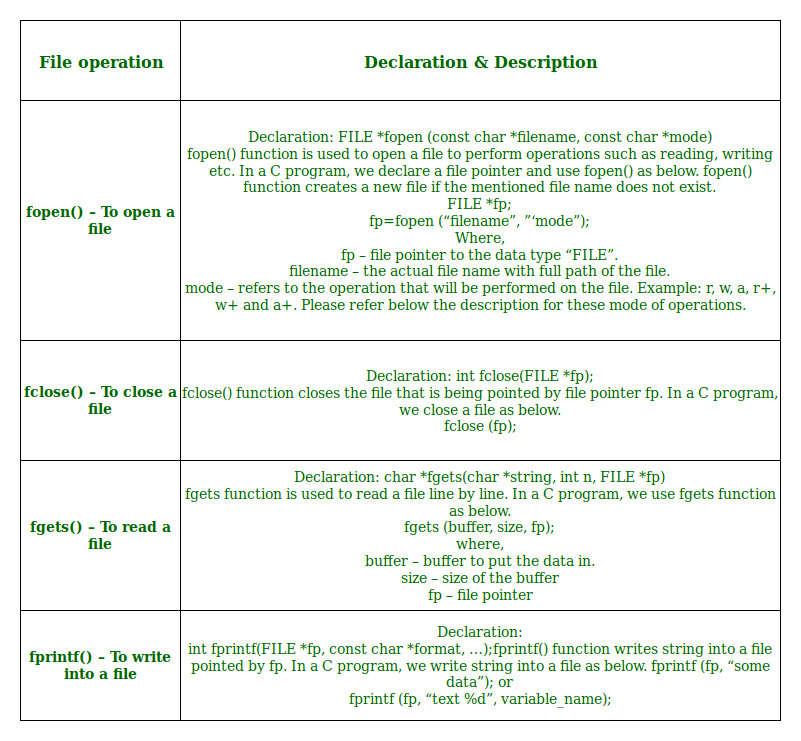My Personal Notes arrow_drop_up